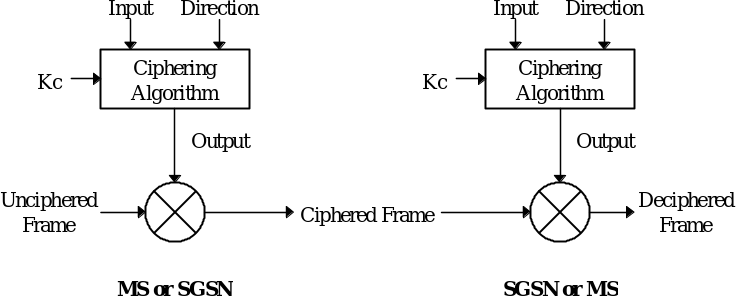## A.2 Ciphering algorithm interface

04.643GPPGeneral Packet Radio Service (GPRS)Mobile Station - Serving GPRS Support Node (MS-SGSN) Logical Link Control (LLC) layer specificationTS

The ciphering algorithm has three input parameters:

– the ciphering key (Kc);

– the frame-dependent input (Input); and

– the transfer direction (Direction).

The ciphering algorithm has one output parameter:

– Output.

The relationship between the input and output parameters and the ciphering algorithm is illustrated in Figure A.1.Figure A.1: GPRS ciphering environment

The input and output parameters and the other elements from Figure A.1 are defined in Table A.1.

Table A.1: Ciphering parameters and frames

 Parameter Length Description Kc 64 bits The LLGMM-ASSIGN-REQ Kc parameter received from GMM. Input 32 bits A modulo counter as defined in subclause A.2.1. Direction 1 bit Set to 0 if the direction of LLC frame transmission is from the MS to the SGSN.Set to 1 if the direction of LLC frame transmission is from the SGSN to the MS. Ciphering Algorithm – A GPRS ciphering algorithm as determined by the LLGMM-ASSIGN-REQ Ciphering Algorithm parameter received from GMM. Output maximum 1 523 octets The output of Ciphering Algorithm. Unciphered Frame maximum 1 523 octets An LLC layer I or UI frame to be ciphered. Ciphered Frame maximum 1 523 octets A ciphered LLC layer I or UI frame. Only the information field and the FCS field shall be ciphered. Ciphered Frame shall be generated by bitwise XOR of Output and the Information Field and FCS Field of Unciphered Frame starting from bit (1, 1). Deciphered Frame maximum 1 523 octets A deciphered LLC layer I or UI frame. Deciphered Frame shall be generated by bitwise XOR of Output and the ciphered part of Ciphered Frame starting from bit (1, 1). When transmitting an LLC frame, Deciphered Frame shall be identical to Unciphered Frame if no transmission errors have occurred.

It is an implementation option to optimise the ciphering algorithm by for example producing only as many Output octets as is needed to cipher Unciphered Frame.

## A.2.1 Generation of Input

The Input parameter shall be generated according to the following algorithm if the frame is a UI frame:

Input = ( ( IOV‑UI  SX ) + LFN + OC ) modulo 232

The Input parameter shall be generated according to the following algorithm if the frame is an I frame:

Input = ( IOV‑I + LFN + OC ) modulo 232

where:

– IOV‑UI is a 32 bit random value generated by the SGSN.

– IOV‑I is a 32 bit random value generated by the SGSN.

– LFN is the LLC frame number in the LLC frame header. LFN is a binary value with a length of nine bits. For I frames, N(S) shall be used as the LFN. For UI frames, N(U) shall be used as the LFN.

– OC is a binary overflow counter that is calculated and maintained independently at the sending and receiving sides. The length of OC is 32 bits. There are four OC counters associated with each DLCI; two for unacknowledged information transfer (one for each direction of transmission), and two for acknowledged information transfer (one for each direction of transmission). An OC for acknowledged operation shall be set to 0 whenever ABM operation is (re‑)established for the corresponding DLCI. OC shall be incremented by 512 every time when the corresponding LFN rolls over, i.e., when LFN exhausts its modulo and restarts counting from 0, so that OC and LFN when added together in effect is a 32 bit modulo 232 counter.

– SX is a 32 bit SAPI XOR mask calculated as follows: SX = 227 • SAPI + 231.

– + is the binary addition operation.

–  is the bitwise XOR operation.

Annex B (informative):
LLC layer states for peer-to-peer operation